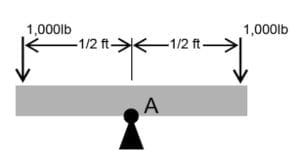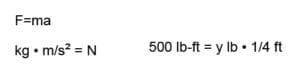# Torque vs. EnergyFor many of us working in the energy industry, the fundamentals of physics have practical and essential applications in our day-to-day operations. There are dozens of different terms and measurements applied during extraction, refinement, and transportation operations, and while some of these are the result of advanced calculations, all of them are based on simple principles that have broad applications in all kinds of environments. So let’s get back to the basics:

### Fundamental Physics Glossary

When it comes to physics or mathematics, the terms force, work, energy, and torque are all defined by specific dimensions and properties. While these concepts can be closely related—and often easily confused—there are several key differences that distinguish them from each other.

Force — Force is pushing or pulling interaction with an object that can alter its movement, shape, or size. According to the International System of Units (SI), it is measured in newtons and is measured in pounds in the English measurement system—1 newton of force is roughly equivalent to about 0.225 pound-force units.

Energy — In mechanics, energy describes the amount of work needed to shift an object to a certain speed in a specific direction. Energy is measured in newton-meters. A single newton-meter is equal to the energy transferred by a one-newton force acting on an object over a one-meter distance. This measurement is called joules in the SI and its equivalent is foot-pounds in the English measurement systems.

Work — Work is a function of force and is calculated by multiplying applied force over a certain distance. Essentially, it describes the transformation of energy into a different form or to a different place. In the SI system, work is measured in joules much like energy.

Torque — Torque is defined and used to describe a type of moment that has a tendency to impart a change in angular momentum (i.e. rotation) of a body about its longitudinal axis. This measurement is defined using Newton-meters or pound-feet rather than joules.

Take a driveshaft sitting on a fulcrum-like seesaw for example—a 1,000-pound force acting at a perpendicular distance of ½-feet from the rotation point, A, causes a 500 lb-ft moment about the rotation point, A. In the physics world, this is referred to as a “bending moment” because it induces bending.Now, apply the same 500 lb-ft moment to the same driveshaft (which has a diameter of 6 inches) about the longitudinal axis. This twists the driveshaft, putting it into “torsion” which is a different phenomenon than bending because of torsion subjects the material to shear stress, instead of normal stress as in bending.Did you get all that? Good—let’s dive a little deeper.

### Torque and Energy: What’s the Real Difference?

It’s easy to confuse torque and energy. Energy comes in many forms—thermal energy, kinetic energy, potential energy, gravitational potential energy, nuclear energy, etc—but none of these are the same as torque.

While torque is technically a type of moment, the two terms shouldn’t be used interchangeably. Torque is specific to the application of force to accomplish rotational rather than directional movement—it’s what you apply to lug nuts when you get a flat tire.

There is one key aspect of torque that has multiple, practical applications in our typical work environment: applied force can be reduced by increasing distance. Using a lever or long handle to rotate an object increases the moment arm of the lever, which linearly increases the distance (or arc length) traveled. This means the lever operator expends less force over a longer distance rather than more force over a shorter distance, which makes the process much easier.

### Significance in the Energy Industry

Torque, energy, and other physics-related terminology are used in almost every aspect of the energy industry. Drilling operations include a wide range of equipment that rely on these principles to function, so it’s important to have a firm grasp on these fundamentals to fully understand the nature and purpose of operations. It’s also good to know the difference between torque and energy, as well as the units used to measure them.

As a premier supplier of energy tools and equipment, Ramey Martin offers a diverse selection of practical and reliable solutions to our clients. We stand behind our products and take pride in the fact that all of them are made right here in the United States.